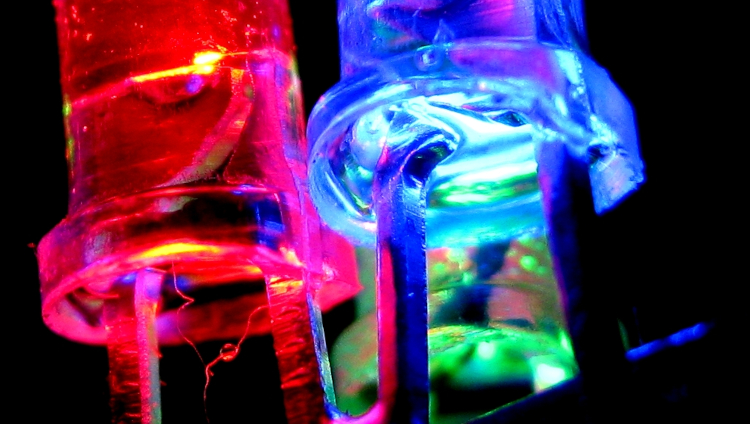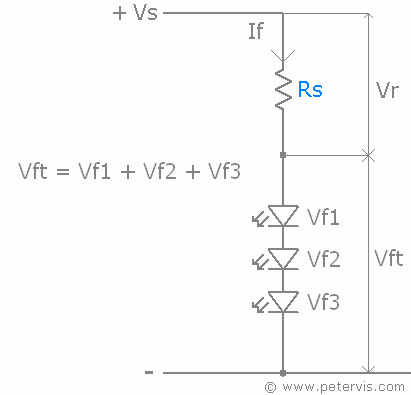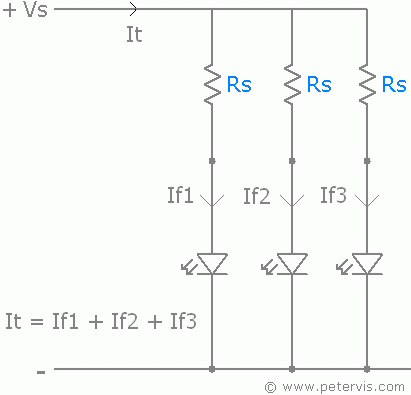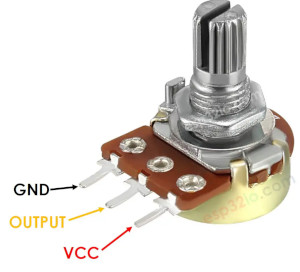# LEDsThe forward current of a LED depends on its color. The regular cheap 3-10 mm LED diodes range from $$1.8-2.2 V$$ (red, orange, yellow, etc) to $$3.2-3.8 V$$ (white, green, warm white, etc). They have typical a forward current of $$20 mA$$, and a maximum forward current of $$30 mA$$1 (for reference, a GPIO pin of an ESP32 provides up to ca max $$40 mA$$).

## Resistance

Since these LEDs all generally have consistent forward currents and forward voltages, we can just use Ohms law and work out a reference table for common supply voltages.

Supply voltage $$V_{s}$$$$V_{f} = 1.8$$$$V_{f} = 3.2 V$$
$$3.3 V$$$$75 Ω$$$$5 Ω$$
$$5 V$$$$160 Ω$$$$90 Ω$$
$$12 V$$$$510 Ω$$$$440 Ω$$

The resistor values are assume the typical forward current of 20 mA.

Forward voltage $$V_{f}$$$$V_{s} = 3.3V$$$$V_{s} = 5 V$$$$V_{s} = 12 V$$
$$1.8 V$$$$75 Ω$$$$160 Ω$$$$510 Ω$$
$$3.2 V$$$$5 Ω$$$$90 Ω$$$$440 Ω$$

The voltage across the circuit is equal to the sum of the voltage across the components in the circuit, the resistor and the LED.

So for an LED witha forwar d voltage $$V_{f} = 1.8V$$ that is connected to a supply voltage $$V_{s} = 3.3V$$, the voltage across the resistor is $$V = V_{s} - V_{f} = 1.5V$$.

>>> I = 0.02     # 20 mA == 0.02 A
>>> Vs = 3.3
>>> Vf = 1.8
>>> V = Vs - Vf
>>> V/I          # R = V/I
75               # 75 Ohm


So to connect LED with a forward voltage of 1.8 V to 3.3 V, it should be protected by a 75 Ω resistor.

## Wiring schematics

SchematicsMultiple LEDs connected in series.Proper way to connect multiple LEDs in paralellel, each LED has its own current limiting resistor.

## Pinout

• The cathode leg ($$-$$ or $$K$$) is the shorter leg (usually $$17 mm$$).
• The anode leg ($$+$$ or $$A$$) is the longer leg (usually $$19 mm$$).

# Potentiometers

PotentiometerPinout of a regular potentiometer.
3

Wikipedia: Light emitting diode